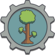tModLoader v2023.10 A mod to make and play Terraria mods
StatModifier Struct Reference

## Public Member Functions

StatModifier (float additive, float multiplicative, float flat=0f, float @base=0f)

float ApplyTo (float baseValue)
Use this to apply the modifiers of this StatModifier to the baseValue . You should assign the value passed in to the return result. For example: More...

StatModifier CombineWith (StatModifier m)

override bool Equals (object obj)

override int GetHashCode ()

StatModifier Scale (float scale)

## Static Public Member Functions

static bool operator!= (StatModifier m1, StatModifier m2)

static StatModifier operator* (float mul, StatModifier m)

static StatModifier operator* (StatModifier m, float mul)
The multiply operator applies a multiplicative effect to the resulting multiplicative modifier. This effect is very rarely used, typical effects use the add operator. More...

static StatModifier operator+ (float add, StatModifier m)

static StatModifier operator+ (StatModifier m, float add)
By using the add operator, the supplied additive modifier is combined with the existing modifiers. For example, adding 0.12f would be equivalent to a typical 12% damage boost. For 99% of effects used in the game, this approach is used. More...

static StatModifier operator- (StatModifier m, float sub)
By using the subtract operator, the supplied subtractive modifier is combined with the existing modifiers. For example, subtracting 0.12f would be equivalent to a typical 12% damage decrease. For 99% of effects used in the game, this approach is used. More...

static StatModifier operator/ (StatModifier m, float div)

static bool operator== (StatModifier m1, StatModifier m2)

## Public Attributes

float Base = 0f
An offset to the base value of the stat. Directly applied to the base stat before multipliers are applied.

float Flat = 0f
Increase to the final value of the stat. Directly added to the stat after multipliers are applied.

## Static Public Attributes

static readonly StatModifier Default = new()

## Properties

float Additive = 1f` [get]`
The combination of all additive multipliers. Starts at 1

float Multiplicative = 1f` [get]`
The combination of all multiplicative multipliers. Starts at 1. Applies 'after' all additive bonuses have been accumulated.

## ◆ ApplyTo()

 float StatModifier.ApplyTo ( float baseValue )

Use this to apply the modifiers of this StatModifier to the baseValue . You should assign the value passed in to the return result. For example:

`damage = CritDamage.ApplyTo(damage)`

could be used to apply a crit damage modifier to a base damage value

For help understanding the meanings of the applied values please make note of documentation for:

The order of operations of the modifiers are as follows:

1. The baseValue is added to Base
2. That result is multiplied by Additive
3. The previous result is then multiplied by Multiplicative
4. Finally, Flat as added to the result of all previous calculations
Parameters
 baseValue The starting value to apply modifiers to
Returns
The result of baseValue after all modifiers are applied

## ◆ operator*()

 static StatModifier StatModifier.operator* ( StatModifier m, float mul )
static

The multiply operator applies a multiplicative effect to the resulting multiplicative modifier. This effect is very rarely used, typical effects use the add operator.

Parameters
 m mul The factor by which the multiplicative modifier is scaled
Returns

## ◆ operator+()

 static StatModifier StatModifier.operator+ ( StatModifier m, float add )
static

By using the add operator, the supplied additive modifier is combined with the existing modifiers. For example, adding 0.12f would be equivalent to a typical 12% damage boost. For 99% of effects used in the game, this approach is used.

Parameters